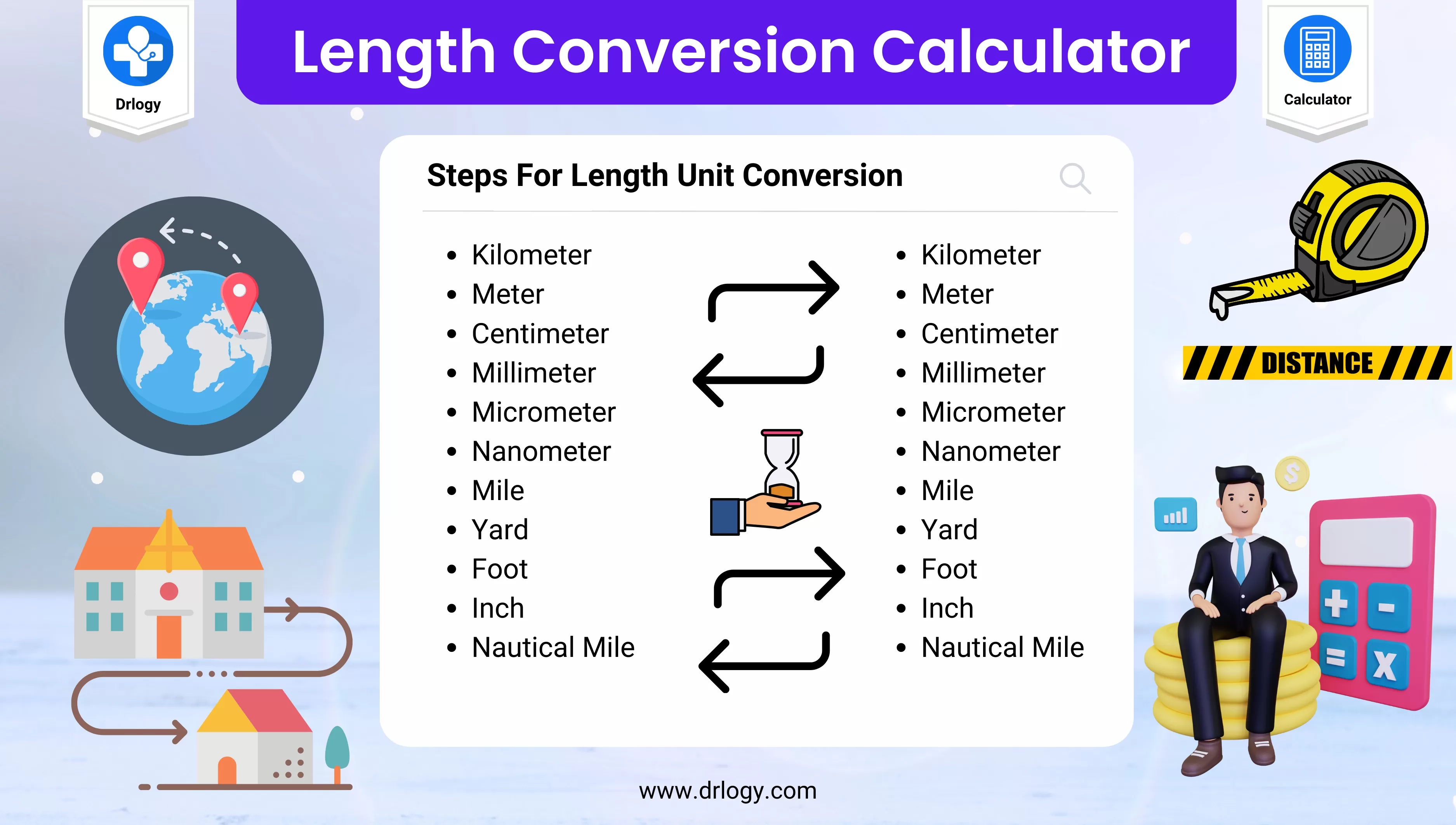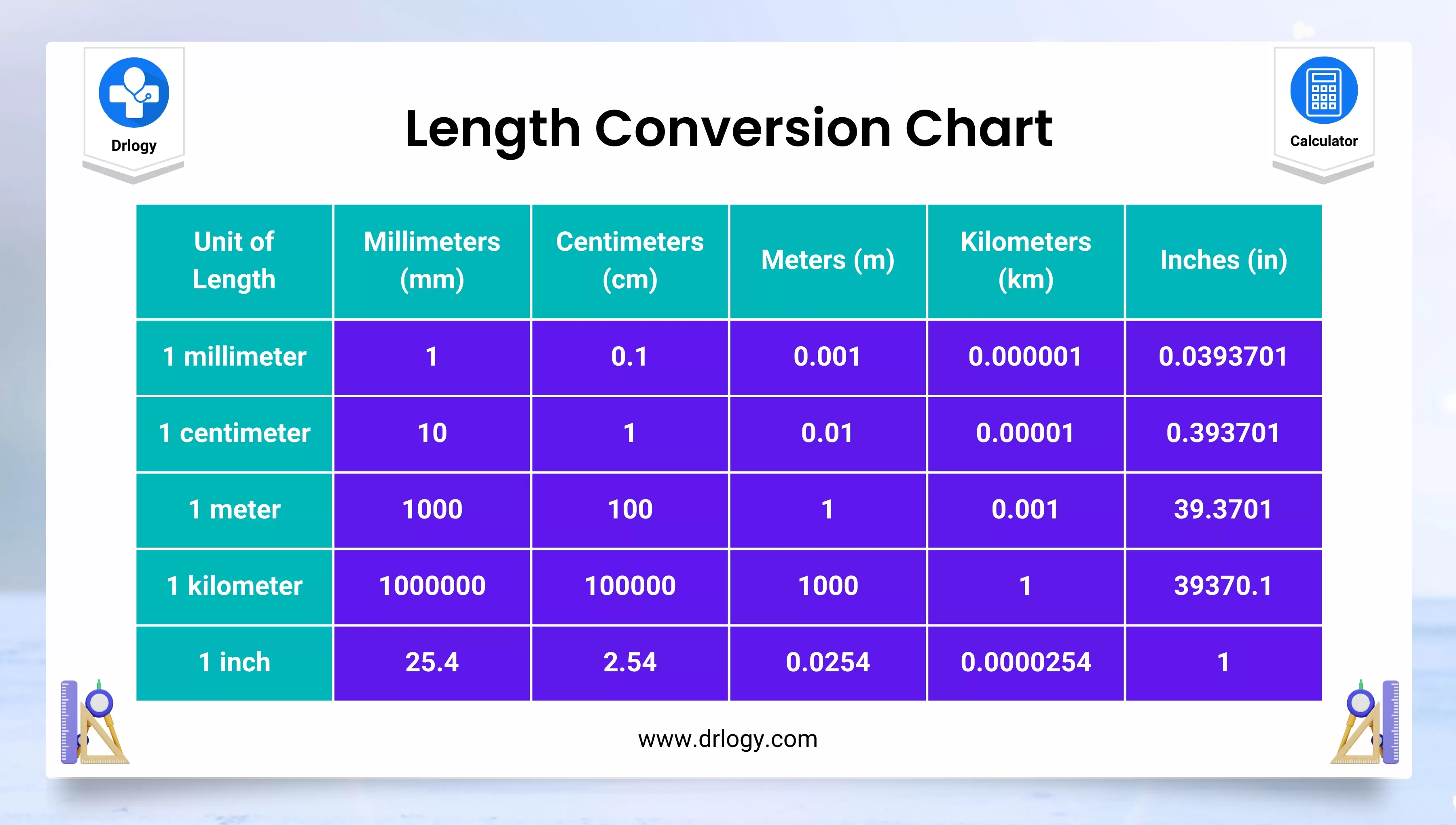• Health
• Pet
• Unit Conversion
• Medical# Length Conversion Calculator With Table And Chart

## Length Conversion Calculator

Result : =
keyboard_arrow_down
=
keyboard_arrow_down

## Length Conversion Calculator Overview

• A length conversion calculator can be used to convert measurements of length from one unit to another Kilometer, Meter, Centimeter, Millimeter, Micrometer, Nanometer, Mile, Yard, Foot, Inch & Mile.

## Steps For Length Conversion Calculator

Here are the steps for using a length conversion calculator:

1. Determine the starting unit: Identify the unit of measurement that you want to convert from, such as meters, feet, or inches.
2. Determine the target unit: Identify the unit of measurement that you want to convert to, such as centimeters, yards, or kilometers.
3. Input the starting value: Enter the starting value for the measurement you want to convert from. For example, if you want to convert 5 meters to feet, input "5" as the starting value.
4. Select the starting unit: Choose the unit of measurement that you are converting from, such as meters.
5. Select the target unit: Choose the unit of measurement that you want to convert to, such as feet.
6. Review the results: Check the output of the calculator to ensure that the conversion has been performed correctly. Make sure the units match the ones you intended to use.
7. Repeat the process as needed: If you need to convert additional measurements, repeat the process using the same calculator and units.

## Length Conversion Chart

Here is a length conversion chart table:

Metric Units Imperial/US Customary Units
1 millimeter (mm) 0.03937 inches (in)
1 centimeter (cm) 0.39370 inches (in)
1 meter (m) 3.28084 feet (ft)
1 meter (m) 39.37008 inches (in)
1 kilometer (km) 0.62137 miles (mi)
1 inch (in) 2.54 centimeters (cm)
1 foot (ft) 0.3048 meters (m)
1 yard (yd) 0.9144 meters (m)
1 mile (mi) 1.60934 kilometers (km)
1 nautical mile (nmi) 1.852 kilometers (km)## Length Conversion

• Length conversion is the process of converting a measurement of length from one unit to another.
• This is commonly used when working with different measurement systems such as metric and imperial units, or when converting measurements to suit specific requirements or standards.
• Length conversion is typically performed using accurate conversion factors. For example, to convert meters to feet, the conversion factor is 3.28084 feet per meter. To convert inches to centimeters, the conversion factor is 2.54 centimeters per inch.
• Common units of measurement for length conversion include millimeters, centimeters, meters, kilometers, inches, feet, yards, and miles. Accurate conversion factors are necessary to ensure accurate results when performing length conversions.
• Length conversion is an important skill in various fields, including construction, engineering, and science.
• It allows for accurate measurements to be taken and provides a common language for communication between professionals working in different measurement systems.

## Measurement Length Conversion Chart

Units of length conversion involve converting a measurement of length from one unit to another. The most common units of length used in conversions are:

### Millimeter (mm)

• The smallest unit of measurement in the metric system, is equivalent to one-thousandth of a meter.

### Centimeter (cm)

• A unit of measurement in the metric system, equivalent to one-hundredth of a meter.

### Meter (m)

• The standard unit of measurement in the metric system, is equivalent to 100 centimeters or 1,000 millimeters.

### Kilometer (km)

• A unit of measurement in the metric system, equivalent to 1,000 meters.

### Inch (in)

• A unit of measurement in the imperial and US customary systems is equivalent to 1/12 of a foot or 2.54 centimeters.

### Foot (ft)

• A unit of measurement in the imperial and US customary systems, equivalent to 12 inches or 0.3048 meters.

### Yard (yd)

• A unit of measurement in the imperial and US customary systems, equivalent to 3 feet or 0.9144 meters.

### Mile (mi)

• A unit of measurement in the imperial and US customary systems is equivalent to 5,280 feet or 1.609 kilometers.

When performing conversions between units of length, it's essential to use accurate conversion factors to ensure accurate results

## Length Conversion Formula

The length conversion formula depends on the units being converted. Here are some common length conversion formulas:

1. Millimeters to Inches: inches = millimeters / 25.4
2. Centimeters to Inches: inches = centimeters / 2.54
3. Meters to Feet: feet = meters / 0.3048
4. Meters to Inches: inches = meters / 0.0254
5. Kilometers to Miles: miles = kilometers / 1.609
6. Inches to Centimeters: centimeters = inches * 2.54
7. Feet to Meters: meters = feet * 0.3048
8. Yards to Meters: meters = yards * 0.9144
9. Miles to Kilometers: kilometers = miles * 1.609
10. Nautical Miles to Kilometers: kilometers = nautical miles * 1.852

These are just some examples of length conversion formulas, and there are many more formulas for converting between different units of length. When performing conversions, it's important to use accurate and reliable conversion factors to ensure accurate results.

## Length Conversion Calculator Benefits

Here are some benefits of using a length conversion calculator:

• Accuracy: A length conversion calculator can perform conversions with high accuracy. It ensures that the conversions are performed using accurate and reliable conversion factors, which reduces the risk of errors.
• Speed: A length conversion calculator can perform calculations quickly, saving time compared to manual conversion methods. This is especially useful when performing multiple conversions or when working with large datasets.
• Ease of Use: A length conversion calculator is easy to use and requires minimal input. Users can simply input the value they want to convert and select the desired units to get an accurate and quick conversion result.
• Accessibility: A length conversion calculator is available online and can be accessed from anywhere, making it convenient for users who need to perform conversions on the go.
• Variety of Units: A length conversion calculator supports a wide range of units of measurement, making it useful for converting between different measurement systems such as metric and imperial units.

Summary

Overall, a length conversion calculator can be a valuable tool for professionals and students in various fields, including engineering, science, and construction, where accurate length conversions are necessary.

Check more unit conversion calculators like this to solve your daily problems on Drlogy Calculator to get the exact solution.

Reference

• List of conversion factors Wikipedia .
• Conversion of Measuring Length .

favorite_border 8596 Likes

## Length Conversion Calculator FAQ

### What are the 7 units of length?

The seven units of length in the International System of Units (SI) are:

1. Meter (m)
2. Kilometer (km)
3. Centimeter (cm)
4. Millimeter (mm)
5. Micrometer (μm)
6. Nanometer (nm)
7. Mile (ml)

These units are used to measure different distances, from very large distances like the size of a city or country to very small distances like the size of atoms and molecules.

### How do you convert inches to cm?

To convert inches to centimeters, you can use the following formula:

Centimeters = Inches x 2.54

So, if you have a measurement of 10 inches, you can convert it to centimeters as follows:

Centimeters = 10 x 2.54 Centimeters = 25.4 cm

Therefore, 10 inches is equal to 25.4 centimeters.

### How do you calculate conversion length?

Generally, to convert from one unit of length to another, you need to:

1. Find the conversion factor between the two units.
2. Multiply the value in the original unit by the conversion factor to get the equivalent value in the new unit.

• For example, to convert meters to centimeters, the conversion factor is 100, because there are 100 centimeters in one meter. To convert centimeters to millimeters, the conversion factor is 10, because there are 10 millimeters in one centimeter.

• To perform the actual calculation, you simply need to multiply the value in the original unit by the conversion factor. For example, to convert 2 meters to centimeters, you would multiply 2 by 100, like this:

2 meters x 100 = 200 centimeters

• Similarly, to convert 50 centimeters to millimeters, you would multiply 50 by 10, like this:

50 centimeters x 10 = 500 millimeters

View More Health

## Medical Calculator

View More Medical

View More Pet

## Unit Conversion Calculator

View More Unit Conversion

## Financial Calculator

View More Financial
• Home >
• Calculator >
• Length Conversion Calculator With Table And Chart
Drlogy®
The Power To Health